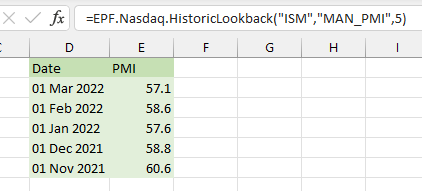## Quandl Excel Formula Reference

The Add-in provides two formulas which are used to populate your spreadsheet with Quandl data. Both of these formulas require you to specify both a Quandl Database Code and a Quandl Dataset Code.

If you haven't done so already please follow the Quandl setup instructions first before using these formulas. Please note the Quandl rate limits, these may affect the frequency you can refresh your Quandl formulas.

#### Quandl Database and Dataset codes

To find the name of the Database and Dataset for the data you are interested in, use the search function on the Quandl website.

For example, here I have searched for one of the "S&P 500 mini" Weekly Commitment of Traders reports from the US Commodity Futures Trading Commission (CFTC):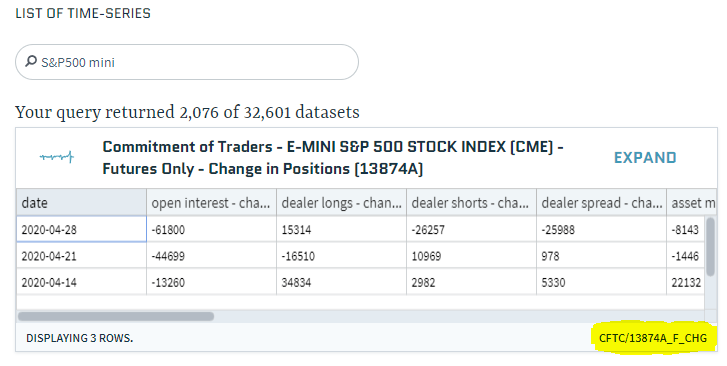Highlighted in yellow is the Database code and the Dataset code separated by a "/", which in this case is:

• Database code: CFTC
• Dataset code: 13874A_F_CHG

If you drill-down on the dataset you can also see this information in the top right corner of the webpage: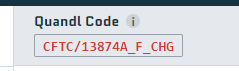#### EPF.Quandl.Last

This formula retrieves a single data point from Quandl. This data point will always be the most recent data point for the specified Quandl database/dataset.

`=EPF.Quandl.Last(databaseCode, datasetCode, field)`

The input parameters required are:

Parameter Description
Database Code The Quandl database code.
Dataset Code The Quandl dataset code.
Field The name of the field.

The section above explains how to find the Database Code and Dataset Code. To find the Field name, simple copy the column header for the data you are interested in. For the example below I would like the "Open Interest - Change" data in my formula: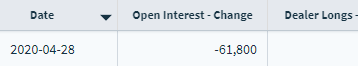Therefore the formula to populate an Excel cell with this number will be:

`=EPF.Quandl.Last("CFTC", "13874A_F_CHG", "Open Interest - Change")`

Which is currently -61800. When the next COT report is released this number will be automatically updated with the new number: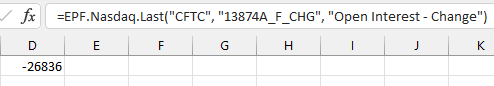Here are some other examples, including commodity prices from CME (Chicago Mercantile Exchange), ICE (Intercontinental Exchange) and GMEX (Minneapolis Grain Exchange):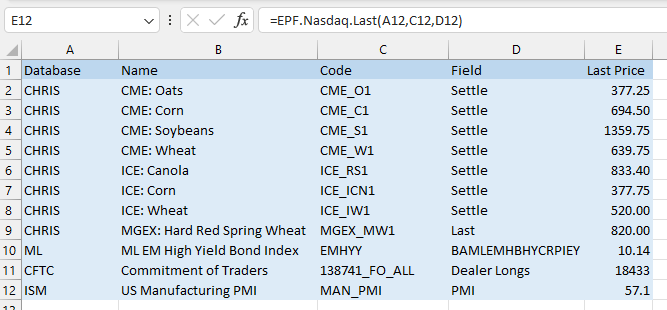#### EPF.Quandl.HistoricLookback

This formula retrieves a series of data points (rows) from Quandl. The data points will always be the most recent data points for the specified Quandl database/dataset.

`=EPF.Quandl.HistoricLookback(databaseCode, datasetCode, numberOfRows)`

The input parameters required are:

Parameter Description
Database Code The Quandl database code.
Dataset Code The Quandl dataset code.
Number of rows The number of rows/periods to return.

For example, to return the last 5 datapoints for the US Manufacturing PMI survey, I will use the following formula:

`=EPF.Quandl.HistoricLookback("ISM","MAN_PMI",5)`